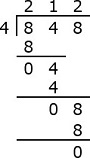# Division without carry

#### Complete Python Prime Pack

9 Courses     2 eBooks

#### Artificial Intelligence & Machine Learning Prime Pack

6 Courses     1 eBooks

#### Java Prime Pack

9 Courses     2 eBooks

### Solution

Step 1

Divide 9 ÷ 3 = 3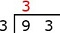Step 2

Multiply 3 × 3 = 9. Subtract 9 – 9.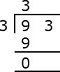Step 3

Bring down 3.Step 4

Divide 3 ÷ 3 = 1.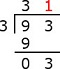Step 5

Multiply 3 × 1 = 3. Subtract 3 – 3 = 0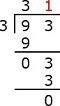### Solution

Step 1

Divide 8 ÷ 4 = 2Step 2

Multiply 4 × 2 = 8. Subtract 8 – 8 = 0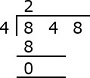Step 3

Bring down 4.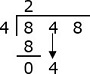Step 4

Divide 4 ÷ 4 = 1.Step 5

Multiply 4 × 1 = 4. Subtract 4 – 4 = 0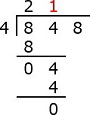Step 6

Bring down 8Step 7

Divide 8 ÷ 4 = 2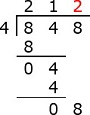Step 8

Multiply 4 × 2 = 8. Subtract 8 – 8 = 0